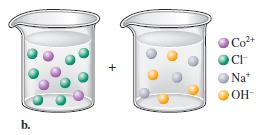# Problem: Write the balanced formula and net ionic equation for the reaction that occurs when the contents of the two beakers are added together. What are the spectator ions in each reaction?

###### FREE Expert Solution
84% (366 ratings)
###### Problem Details

Write the balanced formula and net ionic equation for the reaction that occurs when the contents of the two beakers are added together. What are the spectator ions in each reaction?